# How to optimize a portfolio to manage risk?

4.4Every portfolio has a mix of assets, isn’t it? And if you have a certain amount of money to invest, you will have to distribute this amount across the assets in a portfolio. Think of it like budgeting. Suppose your monthly budget for provision shopping is Rs. 10,000, you will need to allocate a portion of that money to different needs, like staples, vegetables, fruits, spices, and more.

Similarly, when you assign your capital across different assets in a portfolio, the weights for each asset comes into play. For example, let’s take another look at the hypothetical portfolio we’ve been working with, so far.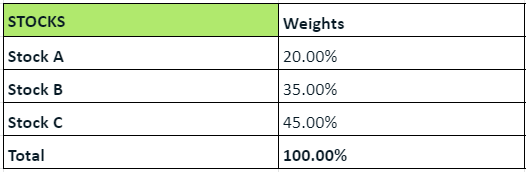For this distribution of assets, we calculated the annual portfolio variance as 89.77%

And the expected annual returns from the portfolio was 326%.

If we change the weight assigned to the stocks a bit, we can reduce the overall portfolio risk. Also, a different combination can increase the risk of the portfolio. What this means is that at one particular combination, the risk of the portfolio will be at the minimum level. This is what we need to find in order to diversify the portfolio and manage its risk.

So, the objective is to find the optimal combination of weights, so the annual portfolio variance is at its minimum. Sounds simple, right? And thanks to an inbuilt tool in MS Excel, making these calculations is also equally simple. This tool is called the Solver (aptly named, isn’t it?).

First, let’s summarize all the important information about our hypothetical portfolio.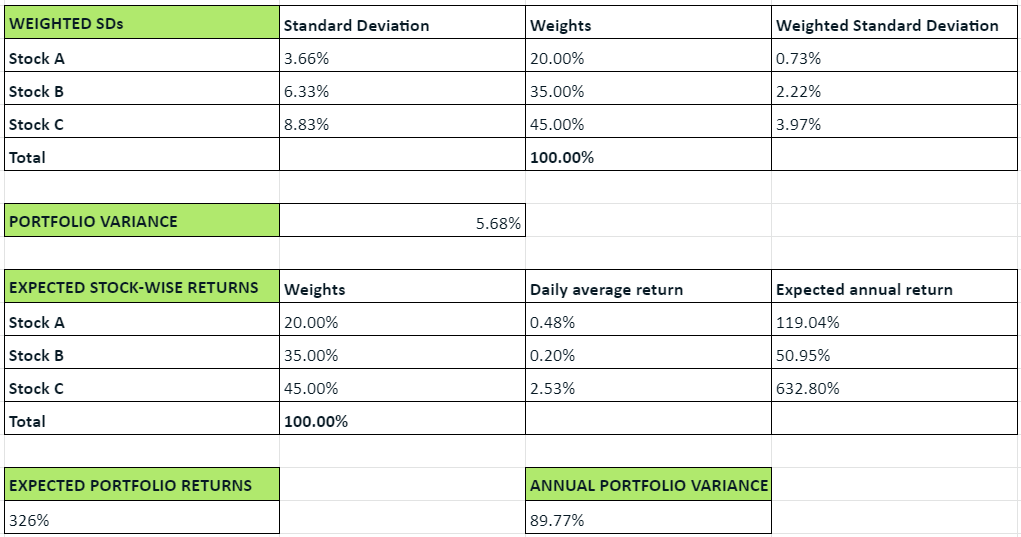### A step-by-step guide to managing risk by modifying the asset allocation

So, here is what we need to do to manage the risk in a portfolio.

1. Set the objective for optimization (in this case, that would be keeping the portfolio risk at the minimum level).
2. Identify the variables that can be changed.
3. Factor in constraints, if any.
4. Arrive at the optimal combination of weights.

Sounds simple? Let’s take a closer look at each step.

### Step 1: Set the objective for optimization

As we mentioned above, in this case, the objective is to keep the portfolio risk at the minimum level. We can do this using the Solver tool, which you’ll find under the ‘Data’ ribbon in Excel.Once you click on that tool, a new box opens up, where you can set the parameters for your problem. The first step is to identify the objective. So, here’s how we do it.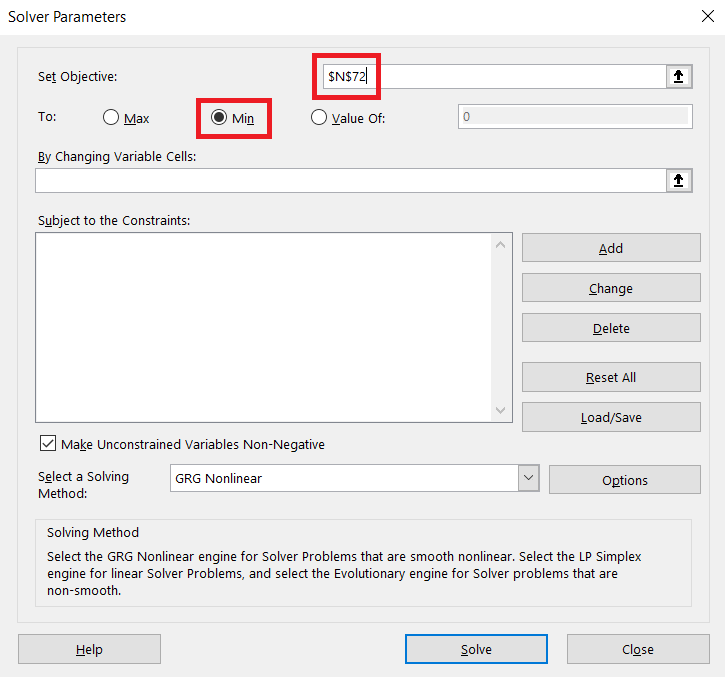Notice how in the tab labeled ‘Set Objective,’ we’ve selected a cell with the address N72? That cell essentially corresponds to the annual portfolio variance in our excel. Currently, this value is at 89.77%. But we want to check if changing the weights of the assets can reduce the variance (and therefore, the risk) even further.

So, after selecting that cell, we choose the objective as ‘Minimum.’ Both the cell and the objective have been highlighted in red boxes.

### Step 2: Identify the variables that can be changed

The variables that we want to change, in this case, are the weights of the assets. Currently, they are as follows:

• Stock A: 20%
• Stock B: 35%
• Stock C: 45%

We can use the Solver to change these variables. Here’s how.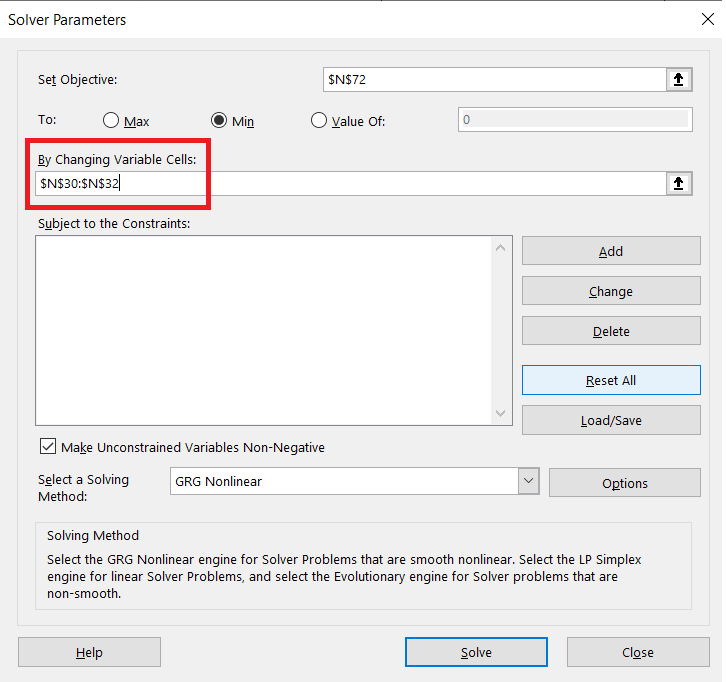The cells highlighted pertain to the weights of the stocks. We want to essentially change them, so the annual portfolio variance is at the minimum level. That is what the parameters we’ve entered so far represent.

### Step 3: Factor in constraints, if any

Constraints here refers to the conditions you may have, if any. One key condition here is that the total of the weights should come up to 100%. Otherwise, if we do not keep that constraint in place, the Solver will have the freedom to reduce the weights to 0%, which would eventually lead to a variance of 0% too - after all, that is the minimum that you can go down to, isn’t it?

It is effectively like saying that if you do not invest at all, your risk will be zero.

But that is not what we’re aiming for. We have a certain amount of capital, and we want to ensure that it is distributed across the stocks in such a way that the overall risk is minimum.

So, here’s how we can factor in this constraint. In the Solver tool, under the section titled ‘Subject to the Constraints,’ select the ‘Add’ option.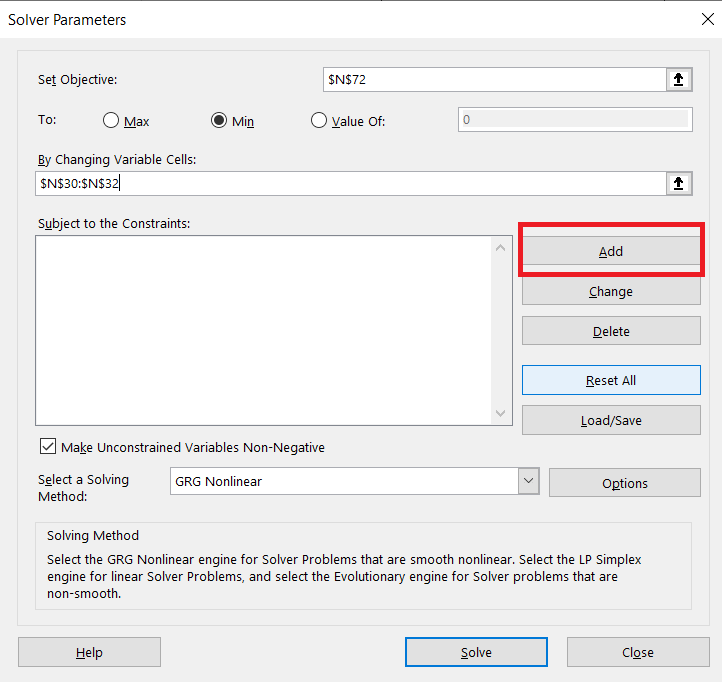Then, add the constraint as follows: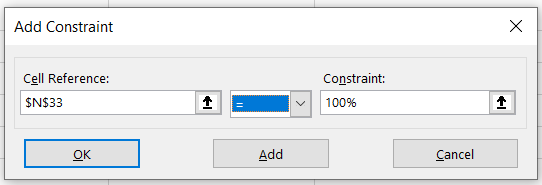Here, cell N33 is the sum total of the weights, and we want that to equal 100%. That’s just what we’ve entered in the tool.

### Step 4: Arrive at the optimal combination of weights

So, now that we’ve submitted all the details needed to solve this requirement, here’s what we have.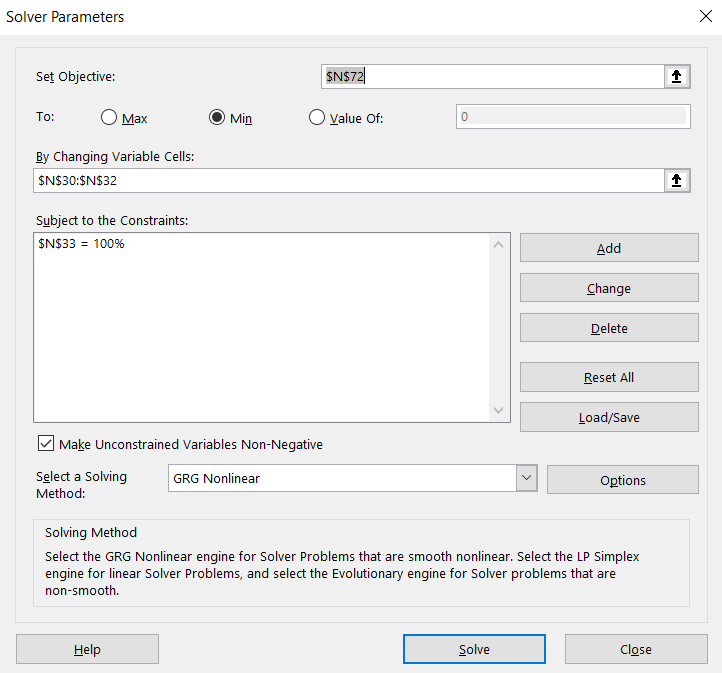This is how you can interpret it, in three parts. We want to:

• Minimize the annual portfolio variance (indicated here by cell N72)
• By changing the weights assigned to the stocks (indicated here by cells N30 to N32)
• And keep the total of the weights at 100% (indicated here by cell N33)

Solving for this set of conditions, this is what we get.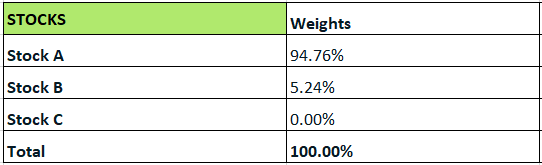And for this scenario, the annual portfolio risk and the annual portfolio returns also change, as follows:See how the portfolio risk falls from 89.77% to 57.64%? This is the minimum risk you can get from our portfolio of the three hypothetical stocks A, B and C. Correspondingly, the returns from the portfolio also drops from 326% to 115%. This ties in well with the low risk-low reward concept.

Now, let’s look at the new weights:

• Stock A: 94.76%
• Stock B: 5.24%%
• Stock C: 0.00%%

Here, stock C’s weight is 0%. That essentially means to reduce the portfolio to its minimum annual variance, you need to choose only stock A and B, with most of the capital being diverted to stock A (nearly 95%). This is theoretically ideal, but practically, if you skew your investments too heavily towards one asset, it defeats the purpose of diversification.

So, what you can do is, in the constraints section of the solver, you can put in a second condition, where the weights of each stock must be greater than 0%.

### Minimum variance portfolio vs. maximum return portfolio

So far, we saw how it is possible to minimize the risk using a different combination of weights. The object was to minimize the variance, so the portfolio we got was the minimum variance portfolio.

Just like this, it is also possible to maximize the returns by modifying the weights. In this case, the risk may go up significantly. That is because the higher the potential returns, the higher the risk too. When you optimize the portfolio’s returns, you get the maximum return portfolio.

#### Wrapping up

Depending on your investment objectives, you can choose to minimize the risk while settling for modest returns, or to maximize the returns even if it means taking on a higher level of risk. But how much money do you stand to lose in any scenario? That is what we’re going to be looking at in the next chapter.

#### A quick recap

• The risk of a portfolio depends on the weights assigned to each asset in it.
• You can optimize the portfolio risk and manage it by modifying the weights of each asset.
• A minimum variance portfolio is where the variance or the risk is the least possible level.
• A maximum return portfolio is where the expected portfolio returns are at the maximum possible level.